Courses
Courses for Kids
Free study material
Offline Centres
More

# Important Questions for CBSE Class 11 Physics Chapter 14 - OscillationsLast updated date: 30th Nov 2023
Total views: 616.5k
Views today: 18.16k

## CBSE Class 11 Physics Chapter-14 Important Questions - Free PDF Download

Free PDF download of Important Questions with solutions for CBSE Class 11 Physics Chapter 14 - Oscillations prepared by expert Physics teachers from latest edition of CBSE(NCERT) books. Register online for Physics tuition on Vedantu.com to score more marks in your Examination.

Also, check CBSE Class 11 Physics Important Questions for other chapters:

 CBSE Class 11 Physics Important Questions Sl.No Chapter No Chapter Name 1 Chapter 1 Physical World 2 Chapter 2 Units and Measurement 3 Chapter 3 Motion in a Straight Line 4 Chapter 4 Motion in a Plane 5 Chapter 5 Law of Motion 6 Chapter 6 Work, Energy and Power 7 Chapter 7 Systems of Particles and Rotational Motion 8 Chapter 8 Gravitation 9 Chapter 9 Mechanical Properties of Solids 10 Chapter 10 Mechanical Properties of Fluids 11 Chapter 11 Thermal Properties of Matter 12 Chapter 12 Thermodynamics 13 Chapter 13 Kinetic Theory 14 Chapter 14 Oscillations 15 Chapter 15 Waves

## Study Important Questions for Class 11 Physics Chapter-14 Oscillations

1 Mark Questions

1. The girl sitting on a swing stands up. What will be the effect on periodic time of swing?

Ans: The time period T is directly proportional to the square root of effective length of pendulum (l). If the girl stands up, the effective length of swing (i.e., pendulum) decreases, thus the time period (T) also decreases.

2. At what distance from the mean position, is the kinetic energy in a simple harmonic oscillator equal to potential energy?

Ans: Consider the displacement of particle executing S.H.M to be Y,

Amplitude of particle executing S.H.M to be a,

Mass of particle to be m,

Angular velocity to be $\omega$.

The kinetic energy will be $\frac{1}{2}m{{\omega }^{2}}\left( {{a}^{2}}-{{y}^{2}} \right)$.

$Potential\text{ }energy=\frac{1}{2}m{{\omega }^{2}}{{y}^{2}}$.

Now, $Kinetic\text{ }energy=Potential\text{ }energy$

$\Rightarrow \frac{1}{2}m{{\omega }^{2}}\left( {{a}^{2}}-{{y}^{2}} \right)=\frac{1}{2}m{{\omega }^{2}}{{y}^{2}}$

$\Rightarrow {{a}^{2}}-{{y}^{2}}={{y}^{2}}$

$\Rightarrow {{a}^{2}}=2{{y}^{2}}$

$\Rightarrow a=\sqrt{2}y$

$\Rightarrow y=\frac{a}{\sqrt{2}}$, which is the required distance from mean position.

3. The soldiers marching on a suspended bridge are advised to go out of steps. Why?

Ans: When the soldiers are marching on a suspended bridge, they are advised to go out of steps. This is because in this case the frequency of marching steps matches with the natural frequency of the suspended bridge. This results in resonance. Thus, amplitude of oscillation increases extensively which may lead to the collapsing of bridges.

4. Is the motion of a simple pendulum strictly simple harmonic?

Ans: The motion of a simple pendulum isn’t strictly harmonic because we make the assumption that $sin\theta =\theta$, which is nearly valid only if $\theta$ is very small.

5. Can a simple pendulum experiment be done inside a satellite?

Ans: Time period of a simple pendulum is given by $T=2\pi \sqrt{\frac{l}{g}}$.

Inside a satellite, the effective value of ‘g’ is $0$. When $g\text{ }=\text{ 0}$,

$T\to \infty$. Thus, the pendulum does not oscillate at all inside the satellite. Hence, the experiment cannot be performed inside a satellite.

6. Give some practical examples of S. H. M?

Ans: Some practical examples of S. H. M. are as follows:

1. Motion of piston in a gas – filled cylinder.

2. Atoms vibrating in a crystal lattice.

3. Motion of helical spring.

7. What is the relation between uniform circular motion and S.H.M?

Ans: Uniform circular motion can be treated as two simple harmonic motions operating at right angles to each other.

8. What is the minimum condition for a system to execute S.H.M?

Ans: The minimum condition for a body to execute S.H.M to have elasticity and inertia.

9. The piston in the cylinder head of a locomotive has a stroke (twice the amplitude) of 1.0 m. If the piston moves with simple harmonic motion with an angular frequency of 200 rad/min, what is its maximum speed?

Ans: In the above question it is given that:

Angular frequency of the piston is $\omega =\text{ }200\text{ }rad/\text{ }min$.

Stroke is $1.0$ m.

Amplitude is $A=\frac{1.0}{2}=0.5$

The maximum speed $\left( {{v}_{\max }} \right)$ of the piston is given by the relation:

${{v}_{\max }}=A\omega$

$=200\times 0.5=100m/\min$.

2 Marks Questions

1. A simple harmonic oscillator is represented by the equation: $Y\text{ }=\text{ }0.40\text{ s}in\left( 440t+0.61 \right)$. Y is in metres t is in seconds. Find the values of 1) Amplitude 2) Angular frequency 3) Frequency of oscillation 4) Time period of oscillation, 5) Initial phase.

Ans: A simple harmonic oscillator is represented by the equation:

$Y\text{ }=\text{ }0.40\text{ }Sin\left( 440t+0.61 \right)$

On comparing with equation of S.H.M: $Y\text{ }=\text{ }asin\left( \omega t+{{\phi }_{0}} \right)$ we get:

1) $Amplitude=0.40m$.

2) $Angular\text{ }frequency=\omega =440Hz$.

3) Frequency of oscillation, $\upsilon =\frac{\omega }{2\pi }=\frac{440}{2\pi }=70Hz$.

4) Time period of oscillation, $T=\frac{1}{\upsilon }=\frac{1}{70}=0.0143s$.

5) $Initial\text{ }phase\left( \phi \right)=0.61rad$.

2. The springs of spring factor k, 2k, k respectively are connected in parallel to a mass m. If the mass = 0.08kg m and k = 2N/m, then find the new time period?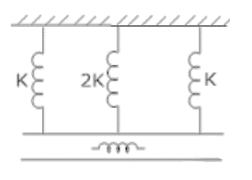Ans: Consider the figure given in the question.

Therefore, total spring constant is given by:

$K'={{K}_{1}}+{{K}_{2}}+{{K}_{3}}$

$=\text{ }K\text{ }+\text{ }2K\text{ }+\text{ }K$

$=\text{ }4K$

$=\text{ }4\text{ }\times \text{ }2\text{ }\left( k\text{ }=\text{ }2\text{ }N/m \right)$

$=8N/m$

Time period is given by:

$T=2\pi \sqrt{\frac{m}{K'}}$

$\Rightarrow T=2\pi \sqrt{\frac{m}{4K}}$

$\Rightarrow T=2\pi \sqrt{\frac{0.08}{4\times 2}}=0.628s$

3. The bob of a vibrating simple pendulum is made of ice. How will the period of swing will change when the ice starts melting?

Ans: The period of swing of simple pendulum will remain unchanged till the location of centre of gravity of the bob left after melting of the ice remains at the fixed position from the point of suspension. When centre of gravity of ice bob after melting is shifted upwards, the effective length of pendulum decreases and thus the time period of swing decreases. Similarly, if centre of gravity shifts downward, time period increases.

4. An 8 kg body performs S.H.M. of amplitude 30 cm. The restoring force is 60N, when the displacement is 30cm. Find: - a) Time period b) the acceleration c) potential and kinetic energy when the displacement is 12cm?

Ans: In the above question it is given that:

$\text{m = 8 kg}$

$\text{a = 30cm = 0}\text{.30m}$

a) $f\text{ }=\text{ }60\text{ }N$,

$Y\text{ }=\text{ }displacement\text{ }=\text{ }0.30m$

$K\text{ }=\text{ }spring\text{ }constant$

Since,

$F\text{ }=\text{ }Ky$

$K=\frac{F}{y}=\frac{60}{0.30}=200N/m$

As, Angular velocity $\omega =\sqrt{\frac{k}{m}}=\sqrt{\frac{200}{8}}=5rad/s$

Time period, $T=\frac{2\pi }{\omega }=\frac{2\times 22}{7\times 5}=1.256s$.

b) $Y\text{ }=\text{ }displacement\text{ }=\text{ }0.12m$

Acceleration, $A={{\omega }^{2}}y$

$A={{\left( 5 \right)}^{2}}\left( 0.12 \right)=3m/{{s}^{2}}$.

$P.E.\text{ }=\text{ }Potential\text{ }energy=\frac{1}{2}k{{y}^{2}}=\frac{1}{2}\left( 200 \right){{\left( 0.12 \right)}^{2}}$

$Potential\text{ }energy=1.44J$

$\text{Kinetic energy = K}\text{.E =}\frac{1}{2}k\left( {{a}^{2}}-{{y}^{2}} \right)$

$\text{K}\text{.E =}\frac{1}{2}\left( 200 \right)\left( {{0.3}^{2}}-{{0.12}^{2}} \right)$

$\text{K}\text{.E =7}\text{.56J}$

5. A particle executing SH.M has a maximum displacement of 4 cm and its acceleration at a distance of 1 cm from its mean position is $3\text{ }cm/{{s}^{2}}$. What will be its velocity when it is at a distance of 2cm from its mean position?

Ans: In the above question it is given that:

Acceleration, $A=3\text{ }cm/{{s}^{2}}$

$Y=1cm$

The acceleration of a particle executing S.H.M is given by:

$A={{\omega }^{2}}Y$

$3={{\omega }^{2}}\left( 1 \right)$

$\omega =\sqrt{3}rad/s$

The velocity of a particle executing S.H.M is given by:

$v=\omega \sqrt{{{a}^{2}}-{{y}^{2}}}$

$v=\sqrt{3}\sqrt{{{4}^{2}}-{{2}^{2}}}$

$v=\sqrt{3}\times \sqrt{12}=6cm/s$

6. What is the ratio of frequencies of the vertical oscillations when two springs of spring constant K are connected in series and then in parallel?

Ans: When two spring of spring constant K are connected in parallel, then effective resistance in

Parallel is given by:

${{K}_{P}}=K+K=2K$

Let ${{f}_{p}}\text{ }=\text{ }frequency\text{ }in\text{ }parallel\text{ }combination$.

${{f}_{p}}=\frac{1}{2\pi }\sqrt{\frac{{{K}_{P}}}{M}}$ …… (1)

In Series combination, effective spring constant for 2 springs of spring constant K is given by:

$\frac{1}{{{K}_{S}}}=\frac{1}{K}+\frac{1}{K}$

$\frac{1}{{{K}_{S}}}=\frac{2}{K}$

${{K}_{S}}=\frac{K}{2}$

Let ${{f}_{s}}\text{ }=\text{ }frequency\text{ }in\text{ }series\text{ }combination$

${{f}_{s}}=\frac{1}{2\pi }\sqrt{\frac{{{K}_{s}}}{M}}$

${{f}_{s}}=\frac{1}{2\pi }\sqrt{\frac{\frac{K}{2}}{M}}$

${{f}_{s}}=\frac{1}{2\pi }\sqrt{\frac{K}{2M}}$ …… (2)

Dividing equation (2) by (1),

$\frac{{{f}_{s}}}{{{f}_{p}}}=\frac{\frac{1}{2\pi }\sqrt{\frac{K}{2M}}}{\frac{1}{2\pi }\sqrt{\frac{{{K}_{P}}}{M}}}$

$\frac{{{f}_{s}}}{{{f}_{p}}}=\frac{1}{2\pi }\sqrt{\frac{K}{2M}}\times 2\pi \sqrt{\frac{M}{{{K}_{P}}}}$

$\frac{{{f}_{s}}}{{{f}_{p}}}=\sqrt{\frac{1}{4}}=\frac{1}{2}$

${{f}_{s}}:{{f}_{p}}=1:2$

7. The kinetic energy of a particle executing S.H.M. is 16J when it is in its mean position. If the amplitude of oscillations is 25cm and the mass of the particle is 5.12kg. Calculate the time period of oscillations?

Ans: In the above question it is given that:

$K.\text{ }E.\text{ }=\text{ }Kinetic\text{ }energy\text{ }=\text{ }16J$.

Now, $m\text{ }=\text{ }Mass\text{ }=\text{ }5.12kg$.

$\omega \text{ }=\text{ }Angular\text{ }frequency$

$a\text{ }=\text{ }amplitude\text{ }=\text{ }25cm\text{ }or\text{ }0.25m$

The Maximum value of K. E. is at mean position is given by:

$\text{K}\text{.E =}\frac{1}{2}m{{\omega }^{2}}{{a}^{2}}$

$\text{16=}\frac{1}{2}m{{\omega }^{2}}{{a}^{2}}$

$\text{32=}m{{\omega }^{2}}{{a}^{2}}$

$\text{32=}\left( 5.12 \right){{\omega }^{2}}{{\left( 0.25 \right)}^{2}}$

${{\omega }^{2}}=\frac{32}{5.12\times {{\left( 0.25 \right)}^{2}}}$

${{\omega }^{2}}=100$

$\Rightarrow \omega =10rad/s$

Now, $T\text{ }=\text{ }Time\text{ }Period=\frac{2\pi }{\omega }=\frac{2\pi }{10}=\frac{\pi }{5}s$.

8. The time period of a body suspended by a spring is T. What will be the new time period if the spring is cut into two equal parts and

1) the body is suspended by one part?

2) suspended by both parts in parallel?

Ans: The time period of oscillation of a body of mass ‘m’ suspended from a spring with force constant ‘k’ is given by:

$T=2\pi \sqrt{\frac{m}{K}}$ …… (1)

1) On cutting the spring in two equal parts, the length of one part is halved and the force constant of each part will be doubled (2k). Therefore, the new time period will be:

${{T}_{1}}=2\pi \sqrt{\frac{m}{2K}}$ …… (2)

From (1) and (2),

${{T}_{1}}=\frac{T}{\sqrt{2}}$

2) If the body is suspended from both parts in parallel, then

the effective force constant of parallel combination $=\text{ }2k\text{ }+\text{ }2k\text{ }=\text{ }4k$.

Therefore, time period will be:

${{T}_{2}}=2\pi \sqrt{\frac{m}{4K}}$ …… (3)

From (1) and (3),

${{T}_{2}}=\frac{T}{2}$

9. A simple pendulum is executing Simple harmonic motion with a time T. If the length of the pendulum is increased by 21 %. Find the increase in its time period?

Ans: The time period of simple pendulum with

$l\text{ }=\text{ }length\text{ }of\text{ }simple\text{ }pendulum$

$g\text{ }=\text{ }acceleration\text{ }due\text{ to }gravity$

Is given by:  $T=2\pi \sqrt{\frac{l}{g}}$

Consider

${{T}_{2}}=\text{ }Final\text{ }time\text{ }period$

${{T}_{1}}=\text{ }Initial\text{ }time\text{ }period$

Therefore,

${{T}_{1}}=2\pi \sqrt{\frac{{{l}_{1}}}{g}}$

${{T}_{2}}=2\pi \sqrt{\frac{{{l}_{2}}}{g}}$

Hence,

$\frac{{{T}_{2}}}{{{T}_{1}}}=\frac{\sqrt{{{l}_{2}}}}{\sqrt{{{l}_{1}}}}$

If ${{l}_{1}}=l$

${{l}_{2}}={{l}_{1}}+{{l}_{1}}\left( \frac{21}{100} \right)$

$\Rightarrow {{l}_{2}}=1.21l$

$\Rightarrow \frac{{{T}_{2}}}{{{T}_{1}}}=\frac{\sqrt{1.21l}}{\sqrt{l}}$

$\Rightarrow \frac{{{T}_{2}}}{{{T}_{1}}}=1.1$

Therefore, percentage increase in time period will be: $\frac{{{T}_{2}}-{{T}_{1}}}{T}\times 100%$

$\Rightarrow \frac{1.1T-T}{T}\times 100%=10%$

10. A particle is executing S H M of amplitude 4 cm and T = 4 sec. find the time taken by it to move from positive extreme position to half of its amplitude?

Ans: Consider

$Y\text{ }=\text{ }displacement$

$t\text{ }=\text{ }time$

$a\text{ }=\text{ }amplitude$

$\omega \text{ }=\text{ }Angular\text{ }frequency$

Now, $Y\text{ }=\text{ }acos\omega t$

Given $Y\text{ }=\frac{a}{2}$

$\Rightarrow \frac{a}{2}=\text{ }acos\omega t$

$\Rightarrow cos\omega t=\frac{1}{2}$

Now, $T\text{ }=\text{ }Time\text{ }Period$

$\omega =\frac{2\pi }{T}$

$\Rightarrow cos\frac{2\pi }{T}t=\frac{1}{2}$

$\Rightarrow cos\frac{2\pi }{4}t=\frac{1}{2}$

Let ${{W}_{A}}$ is work done by spring A & ${{k}_{A\text{ }}}=\text{ }Spring\text{ }Constant$ and

${{W}_{B}}$ is work done by spring B & ${{k}_{B}}\text{ }=\text{ }Spring\text{ }Constant$.

$\frac{{{W}_{A}}}{{{W}_{B}}}=\frac{{{k}_{A}}}{{{k}_{B}}}=\frac{1}{3}$

${{W}_{A}}:{{W}_{B}}=1:3$

11. Two linear simple harmonic motions of equal amplitudes and angular frequency $\omega$ and $2\omega$ are impressed on a particle along axis X and Y respectively. If the initial phase difference between them is $\frac{\pi }{2}$ , find the resultant path followed by the particle?

Ans: Consider

$s\text{ }=\text{ }amplitude\text{ }of\text{ }each\text{ }S.H.M.$

$\omega \text{ }=\text{ }Angular\text{ }frequency$

$t\text{ }=\text{ }time$

Then,

$x=a\sin \omega t$ …… (1)

$y=a\sin \left( 200t+\frac{\pi }{2} \right)$

Where,

x is the displacement along x-axis and y along y-axis.

$y=a\sin 2\omega t$ $\left[ \because \sin \left( \frac{\pi }{2}+\theta \right)=\cos \theta \right]$

Now, $\cos 2\theta =1-2{{\sin }^{2}}\theta$

$y=a\left( 1-2{{\sin }^{2}}\omega t \right)$ …… (2)

Form equation (1),

$\sin \omega t=\frac{x}{a}$

Substituting in eq (2)

$y=a\left( 1-2\frac{{{x}^{2}}}{{{a}^{2}}} \right)$

$y=a-\frac{2{{x}^{2}}}{a}$

$\frac{2{{x}^{2}}}{a}=a-y$

${{x}^{2}}=\frac{a}{2}\left( a-y \right)$

${{x}^{2}}=\frac{{{a}^{2}}}{2}-\frac{ay}{2}$, which represents the resultant path.

12. The acceleration due to gravity on the surface of moon is $1.7\text{ }m/{{s}^{2}}$. What is the time period of simple pendulum on moon if its time period on the earth is 3.5s?

Ans: Consider

$l\text{ }=\text{ }length\text{ }of\text{ }simple\text{ }pendulum$,

$T\text{ }=\text{ }Time\text{ }Period$

$g\text{ }=\text{ }Acceleration\text{ }due\text{ }to\text{ }gravity$.

On earth, $T=2\pi \sqrt{\frac{l}{g}}$ …… (1)

On moon, ${{T}_{1}}=2\pi \sqrt{\frac{l}{g}}$ …… (2)

${{g}_{1}}=Acceleration\text{ }due\text{ }to\text{ }gravity\text{ }on\text{ }moon=1.7\text{ }m/{{s}^{2}}$

$g=Acceleration\text{ }due\text{ }to\text{ }gravity\text{ }on\text{ }earth=9.8m/{{s}^{2}}$

Dividing equation (2) by (1) we get:

$\frac{{{T}_{1}}}{T}=\sqrt{\frac{g}{{{g}_{1}}}}$

$\Rightarrow \frac{{{T}_{1}}}{T}=\sqrt{\frac{9.8}{1.7}}$

$\Rightarrow {{T}_{1}}=T\sqrt{\frac{9.8}{1.7}}=3.5\times \sqrt{\frac{9.8}{1.7}}=8.4s$

13. Using the correspondence of S. H. M. and uniform circular motion, find displacement, velocity, amplitude, time period and frequency of a particle executing SH.M?

Ans: Initially at $t\text{ }=\text{ }0$ the particle is at D, then at $time\text{ }=\text{ }t$ the particle is at point P.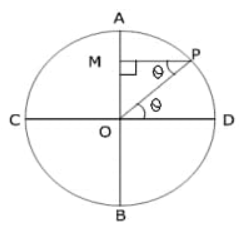Then, drop a perpendicular from P on AB,

If the displacement $OM\text{ }=\text{ }Y$

$Ratios\text{ }of\text{ }circle\text{ }of\text{ }reference\text{ }=\text{ }Amplitude\text{ }=\text{ }a$

Then,

In $\Delta OPM,\text{ }\angle POD\text{ }=\text{ }\angle OPM$ $\left( \because \text{ }Alternate\text{ }Angles \right)$

$\sin \theta =\frac{OM}{OP}$

$\sin \theta =\frac{y}{a}$

$y=a\sin \theta$

Now, $\omega \text{ }=\text{ }Angular\text{ }speed$

$\text{t }=\text{ }time$

Then $\theta =\omega t$

$\Rightarrow y=a\sin \omega t$

Therefore, $\sin \omega t=\frac{y}{a}$ …… (1)

We know that:

$v=\frac{dy}{dt}$

$v=\frac{d\left( a\sin \omega t \right)}{dt}$

$v=a\omega \cos \omega t$

$v=a\omega \sqrt{1-{{\sin }^{2}}\omega t}$ $\left( \because {{\sin }^{2}}t+{{\cos }^{2}}t=1 \right)$

$v=a\omega \sqrt{1-\frac{{{y}^{2}}}{{{a}^{2}}}}$ …… (from 1)

$v=\omega \sqrt{{{a}^{2}}-{{y}^{2}}}$

Now, $A=\frac{dv}{dt}$

Hence, $A=\frac{d\left( a\omega \cos \omega t \right)}{dt}$

$A=-a{{\omega }^{2}}\sin \omega t$

As, $y=a\sin \theta$

Hence, $A=-{{\omega }^{2}}y$

The acceleration is proportional to the negative of displacement is the characteristics of S.H.M.

Time period is given by: $T=\frac{2\pi }{\omega }$.

And frequency is given by: $f=\frac{1}{T}=\frac{\omega }{2\pi }$.

14. A particle executing S.H.M. along a straight line has a velocity of 4m/s when its displacement from mean position is 3 m and 3 m / s when displacement is 4m. Find the time taken to travel 2.5 m from the positive extremity of its oscillation?

Ans: In the above question it is given that:

When, $velocity\text{ }=\text{ }{{v}_{1}}\text{ }=\text{ 4 }m/s$

Then, $displacement\text{ }={{y}_{1}}\text{= }3m$

And when $velocity\text{ }=\text{ }{{v}_{2}}\text{ }=\text{ 3 }m/s$

Then, $displacement\text{ }={{y}_{2}}\text{= 4}m$

Now, ${{v}^{2}}={{\omega }^{2}}\left( {{a}^{2}}-{{y}^{2}} \right)$

Therefore, for case 1:

${{v}_{1}}^{2}={{\omega }^{2}}\left( {{a}^{2}}-{{y}_{1}}^{2} \right)$

$16={{\omega }^{2}}\left( {{a}^{2}}-9 \right)$ …… (1)

And for case 2:

${{v}_{2}}^{2}={{\omega }^{2}}\left( {{a}^{2}}-{{y}_{2}}^{2} \right)$

$9={{\omega }^{2}}\left( {{a}^{2}}-16 \right)$ …… (2)

Dividing eq (1) by (2),

$\frac{16}{9}=\frac{{{\omega }^{2}}\left( {{a}^{2}}-9 \right)}{{{\omega }^{2}}\left( {{a}^{2}}-16 \right)}$

$16\left( {{a}^{2}}-16 \right)=9\left( {{a}^{2}}-9 \right)$

$16{{a}^{2}}-256=9{{a}^{2}}-81$

$7{{a}^{2}}=175$

${{a}^{2}}=\frac{175}{7}$

$a=\sqrt{\frac{175}{7}}=\sqrt{25}$

$a=5m$

${{v}_{1}}^{2}={{\omega }^{2}}\left( {{a}^{2}}-{{y}_{1}}^{2} \right)$

$16={{\omega }^{2}}\left( 25-9 \right)$

$16={{\omega }^{2}}\left( 16 \right)$

$\Rightarrow {{\omega }^{2}}=1$$\Rightarrow \omega =1rad/s When the particle is $2.5m$ from the positive extreme position, its displacement from the mean position, $y\text{ }=\text{ }5\text{ }\text{ }2.5\text{ }=\text{ }2.5m$ . Since the time is measured when the particle is at extreme position: y=a\cos \omega t 2.5=5\cos t \cos t=\frac{1}{2} \Rightarrow t=\frac{\pi }{3}$$\Rightarrow t=1.047s$

15. Springs of spring constants K, 2K, 4K, K ----- are connected in series. A mass M Kg is attached to the lower end of the last spring and the system is allowed to vibrate. What is the time period of oscillation?

Ans: The effective resistance of spring connected in series of individual spring constant ${{k}_{1}},\text{ }{{k}_{2}},\text{ }------\text{ }{{k}_{n}}$ is given by:

$\frac{1}{{{k}_{eff}}}=\frac{1}{{{k}_{1}}}+\frac{1}{{{k}_{2}}}+\frac{1}{{{k}_{3}}}+........+\frac{1}{{{k}_{n}}}$

Now, ${{k}_{1}}=k,\text{ }{{k}_{2}}=2k,{{k}_{3}}=8k,...$

Therefore,

$\frac{1}{{{k}_{eff}}}=\frac{1}{k}+\frac{1}{2k}+\frac{1}{4k}+\frac{1}{8k}+...$

$\frac{1}{{{k}_{eff}}}=\frac{1}{k}\left[ 1+\frac{1}{2}+\frac{1}{4}+\frac{1}{8}+... \right]$

Now sum of infinite G.P. $=\frac{a}{1-r}$

$\frac{1}{{{k}_{eff}}}=\frac{1}{k}\left[ \frac{1}{1-\frac{1}{2}} \right]$

${{k}_{eff}}=\frac{k}{2}$

Now time period is given by: $T=2\pi \sqrt{\frac{M}{{{k}_{eff}}}}$

$\Rightarrow T=2\pi \sqrt{\frac{2M}{k}}$

16. A particle is moving with SHM in a straight line. When the distance of the particle from mean position has values ${{\mathbf{x}}_{\mathbf{1}}}$ and ${{\mathbf{x}}_{\mathbf{2}}}$ the corresponding values of velocities are ${{\mathbf{v}}_{1}}$ and ${{\mathbf{v}}_{\mathbf{2}}}$. Show that the time period of oscillation is given by:

$T=2\pi {{\left[ \frac{{{x}_{2}}^{2}-{{x}_{1}}^{2}}{{{v}_{1}}^{2}-{{v}_{2}}^{2}} \right]}^{\frac{1}{2}}}$

Ans: If

$a\text{ }=\text{ }amplitude$;

$y\text{ }=\text{ }displacement$;

$w\text{ }=\text{ }angular\text{ }frequency$,

$v\text{ }=\text{ }Velocity$, then

${{v}^{2}}={{\omega }^{2}}\left( {{a}^{2}}-{{x}^{2}} \right)$

Thus,

For case 1: ${{v}_{1}}^{2}={{\omega }^{2}}\left( {{a}^{2}}-{{x}_{1}}^{2} \right)$ ….... (1)

For case 2: ${{v}_{2}}^{2}={{\omega }^{2}}\left( {{a}^{2}}-{{x}_{2}}^{2} \right)$ …… (2)

Subtracting equation (2) from equation (1),

${{v}_{1}}^{2}-{{v}_{2}}^{2}={{\omega }^{2}}\left( {{a}^{2}}-{{x}_{1}}^{2} \right)-{{\omega }^{2}}\left( {{a}^{2}}-{{x}_{2}}^{2} \right)$

${{v}_{1}}^{2}-{{v}_{2}}^{2}={{\omega }^{2}}{{a}^{2}}-{{\omega }^{2}}{{x}_{1}}^{2}-{{\omega }^{2}}{{a}^{2}}+{{\omega }^{2}}{{x}_{2}}^{2}$

${{v}_{1}}^{2}-{{v}_{2}}^{2}={{\omega }^{2}}\left( {{x}_{2}}^{2}-{{x}_{1}}^{2} \right)$

${{\omega }^{2}}=\frac{{{v}_{1}}^{2}-{{v}_{2}}^{2}}{{{x}_{2}}^{2}-{{x}_{1}}^{2}}$

$\omega =\sqrt{\frac{{{v}_{1}}^{2}-{{v}_{2}}^{2}}{{{x}_{2}}^{2}-{{x}_{1}}^{2}}}$

Thus,

$T=2\pi {{\left[ \frac{{{x}_{2}}^{2}-{{x}_{1}}^{2}}{{{v}_{1}}^{2}-{{v}_{2}}^{2}} \right]}^{\frac{1}{2}}}$

17. A circular disc of mass 10 kg is suspended by a wire attached to its centre. The wire is twisted by rotating the disc and released. The period of torsional oscillations is found to be 1.5 s. The radius of the disc is 15 cm. Determine the torsional spring constant of the wire. (Torsional spring constant α is defined by the relation $J=-\alpha \theta$. where J is the restoring couple and $\theta$ the angle of twist)

Ans: In the above question it given that:

$Mass\text{ }of\text{ }the\text{ }circular\text{ }disc,\text{ }m\text{ }=\text{ }10\text{ }kg$

$Radius\text{ }of\text{ }the\text{ }disc,\text{ }r\text{ }=\text{ }15\text{ }cm\text{ }=\text{ }0.15\text{ }m$

$The\text{ }torsional\text{ }oscillations\text{ }of\text{ }the\text{ }disc\text{ }has\text{ }a\text{ }time\text{ }period,\text{ }T\text{ }=\text{ }1.5\text{ }s$

The moment of inertia of the disc is:

$I=1/2m{{r}^{2}}$

$=1/2\left( 10 \right){{\left( 0.15 \right)}^{2}}$

$=0.1125kg/{{m}^{2}}$

Time period,

$T=2\pi \sqrt{\frac{I}{\alpha }}$

And

$\alpha =4{{\pi }^{2}}I/{{T}^{2}}$

$=4{{\pi }^{2}}\left( \frac{0.1125}{{{1.5}^{2}}} \right)$

$=1.972N/rad$

Therefore, the torsional spring constant of the wire is $1.972N/rad$.

3 Marks Questions

1. A mass = m suspended separately from two springs of spring constant ${{\mathbf{k}}_{\mathbf{1}}}$and ${{\mathbf{k}}_{\mathbf{2}}}$ gives time period ${{\mathbf{t}}_{\mathbf{1}}}$ and ${{\mathbf{t}}_{\mathbf{2}}}$ respectively. If the same mass is connected to both the springs as shown in figure. Calculate the time period of the combined system?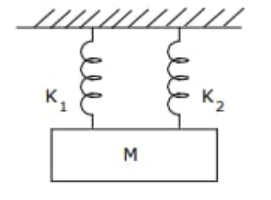Ans: If

$T\text{ }=\text{ }Time\text{ }Period\text{ }of\text{ }simple\text{ }pendulum$

$m\text{ }=\text{ }Mass$

$k\text{ }=\text{ }Spring\text{ }constant$

Then, $T=2\pi \sqrt{\frac{m}{k}}$

And $k=\frac{4{{\pi }^{2}}m}{{{T}^{2}}}$

Thus,

For 1st spring: ${{k}_{1}}=\frac{4{{\pi }^{2}}m}{{{T}_{1}}^{2}}$

For 2nd spring: ${{k}_{2}}=\frac{4{{\pi }^{2}}m}{{{T}_{2}}^{2}}$

When springs is connected in parallel, effective spring constant is given by:

$k={{k}_{1}}+{{k}_{2}}$

$\frac{4{{\pi }^{2}}m}{{{T}^{2}}}=\frac{4{{\pi }^{2}}m}{{{T}_{1}}^{2}}+\frac{4{{\pi }^{2}}m}{{{T}_{2}}^{2}}$

$\Rightarrow \frac{1}{{{T}^{2}}}=\frac{1}{{{T}_{1}}^{2}}+\frac{1}{{{T}_{2}}^{2}}$, where T is the time period of the combined system.

2. Show that the total energy of a body executing SHM is independent of time.

Ans: Let

$y\text{ }=\text{ }displacement\text{ }at\text{ }any\text{ }timet$

$a\text{ }=\text{ }amplitude$

$\omega \text{ }=\text{ }Angular\text{ }frequency$

$v\text{ }=\text{ }velocity$,

$y\text{ }=\text{ }asin\omega t$

Therefore,

$v=\frac{dy}{dt}$

$v=\frac{d\left( asin\omega t \right)}{dt}$

$\text{v }=\text{ }a\omega cos\omega t$

Now, $kinetic\text{ }energy=K.E=\frac{1}{2}m{{v}^{2}}$

$\Rightarrow K.E=\frac{1}{2}m{{a}^{2}}{{\omega }^{2}}co{{s}^{2}}\omega t$ …… (1)

$Potential\text{ }energy\text{ }=\frac{1}{2}k{{y}^{2}}$

$P.E.=\frac{1}{2}k{{a}^{2}}si{{n}^{2}}\omega t$ …… (2)

$\text{total energy}=K.E+P.E=\frac{1}{2}m{{a}^{2}}{{\omega }^{2}}co{{s}^{2}}\omega t+\frac{1}{2}k{{a}^{2}}si{{n}^{2}}\omega t$

Now, $\omega =\sqrt{\frac{k}{m}}$

$k={{\omega }^{2}}m$

$\text{total energy}=\frac{1}{2}k{{a}^{2}}co{{s}^{2}}\omega t+\frac{1}{2}k{{a}^{2}}si{{n}^{2}}\omega t$

$\text{total energy}=\frac{1}{2}k{{a}^{2}}\left( si{{n}^{2}}\omega t+co{{s}^{2}}\omega t \right)$

$\text{total energy}=\frac{1}{2}k{{a}^{2}}$

Thus, total mechanical energy is always constant is equal to $\frac{1}{2}k{{a}^{2}}$. The total energy is independent of time. The potential energy oscillates with time and has a maximum value of $\frac{1}{2}k{{a}^{2}}$.

Similarly, K. E. oscillates with time and has a maximum value of $\frac{1}{2}k{{a}^{2}}$. At any instant total energy is constant i.er. $\frac{1}{2}k{{a}^{2}}$.

3. A particles moves such that its acceleration ‘a’ is given by a = -b x where x = displacement from equilibrium position and b is a constant. Find the period of oscillation.

Ans: In the above question it is given that $a\text{ }=\text{ }-b\text{ }x$.

Since $a\propto x$ and is directed opposite to x, the particle moves in S.H. M.

If we consider only magnitude $a\text{ }=\text{ }b\text{ }x$

Or $\frac{x}{a}=\frac{1}{b}$

Thus, $\frac{displacement}{acceleration}=\frac{1}{b}$ …… (1)

Time period, $T=2\pi \sqrt{\frac{displacement}{acceleration}}$

Therefore, $T=2\pi \sqrt{\frac{1}{b}}$

4. A particle is S.H.M. is described by the displacement function:

$x=A\cos \left( \omega t+\phi \right),\omega =\frac{2\pi }{T}$

If the initial (t=0) position of the particle is 1cm and its initial velocity is, $\pi cm/s$, What are its amplitude and phase angle?

Ans: At $t=0$, $x=1cm$, $\omega =\pi cm/s$ and $v=\pi cm/s$

A particle is S.H.N. is described by the displacement function:

$x=A\cos \left( \omega t+\phi \right)$$\Rightarrow 1=A\cos \phi v=\frac{dx}{dt} v=\frac{d\left( A\cos \left( \omega t+\phi \right) \right)}{dt} v=-A\omega \sin \left( \omega t+\phi \right) \pi =-A\pi \sin \phi -1=A\sin \phi …… (2) Squaring (10 and (2) and adding, {{A}^{2}}{{\cos }^{2}}\phi +{{A}^{2}}{{\sin }^{2}}\phi =2 {{A}^{2}}=2 A=\sqrt{2}cm, which is the required amplitude. Dividing (1) by (2) we get, $\left( \frac{A\sin \phi }{Aco\operatorname{s}\phi } \right)=-1$ $\tan \phi =-1$ \phi =\frac{3\pi }{4}, which is the required phase angle. 5. Determine the time period of a simple pendulum of length = l when mass of bob = m kg. Ans: A simple pendulum consists of a heavy point mass body suspended by a weightless inextensible and perfectly flexible string from a rigid support which is free to oscillate. The distance between the point of suspension and the point of oscillation is the effective length of the pendulum. $M\text{ }=\text{ }Mass\text{ }of\text{ }Bob$ $x\text{ }=\text{ }Displacement\text{ }=\text{ }OB$ $l\text{ }=\text{ }length\text{ }of\text{ }simple\text{ }pendulum$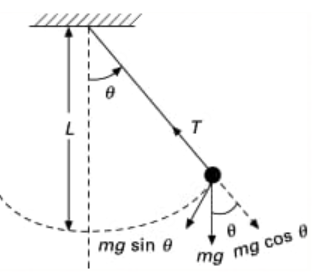Let the bob is displaced through a small angle θ the forces acting on it:- 1) $weight\text{ }=\text{ }Mg\text{ }acting\text{ }vertically\text{ }downwards$. 2) $Tension\text{ }=\text{ }T$ acting upwards. Dividing Mg into its components $mgcos\text{ }\theta \text{ }\And \text{ m}gsin\text{ }\theta$ $T\text{ }=\text{ m}gcos\text{ }\theta$ $F\text{ }=\text{ m}gsin\text{ }\theta$ - ve sign shows force is divested towards the ocean positions. If $\theta$ is Small, \sin \theta \approx \theta =\frac{A\text{rc OB}}{l}=\frac{x}{l} F=-mg\frac{x}{l} In S.H.M restoring force, -mg\sin \theta =-mg\theta =-\frac{mgx}{l} When, k=spring constant F=-kx -\frac{mgx}{l}=-kx k=\frac{mg}{l} T=2\pi \sqrt{\frac{m}{k}} T=2\pi \sqrt{\frac{ml}{mg}} T=2\pi \sqrt{\frac{l}{g}} Thus, 1) Time period depends on the length of pendulum and ‘g’ of place where experiment is done. 2) T is independent of the amplitude of vibration provided and it is small and also of the mass of bob. 6. Which of the following examples represent periodic motion? a) A swimmer completing one (return) trip from one bank of a river to the other and back. Ans: As motion of the swimmer between the banks of the river is to and fro, it does not have a definite period. The time taken by the swimmer during his back-and-forth journey may not be the same. Hence, the swimmer's motion is not periodic. b) A freely suspended bar magnet displaced from its N-S direction and released. Ans: If a magnet is displaced from its N-S direction and released, then the motion of the freely-suspended magnet is periodic. This is because the magnet oscillates about its position with a definite period of time. c) A hydrogen molecule rotating about its centre of mass. Ans: If we consider a hydrogen molecule rotating about its centre of mass, it is observed that it comes to the same position after an equal interval of time. This type of motion is periodic motion. d) An arrow released from a bow. Ans: When an arrow is released from a bow, it moves only in the forward direction. There is no motion repeated in equal intervals of time. Therefore, this motion is not periodic. 7. Figure 14.27 depicts four x-t plots for linear motion of a particle. Which of the plots represent periodic motion? What is the period of motion (in case of periodic motion)? a)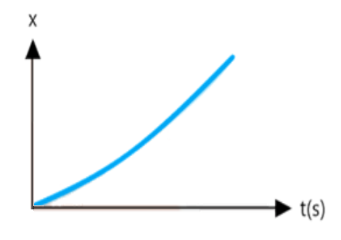Ans: Graph in figure (a) is not a periodic motion. It is a unidirectional, linear uniform motion. Also, there is no repetition of motion in this case. b)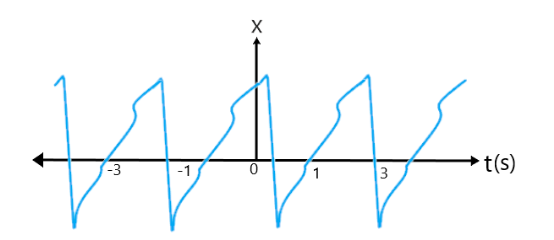Ans: Graph in figure (b) is a periodic motion. The motion of the particle repeats itself after 2s. Thus, it is a periodic motion with a period of 2s. c)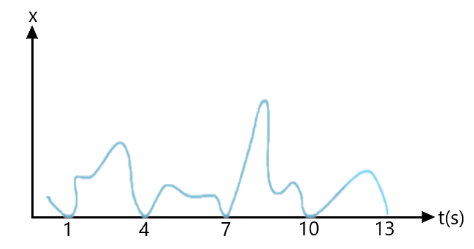Ans: Graph in figure (c) is not a periodic motion as the particle repeats the motion in one position only. For a periodic motion, the entire motion of the particle must be repeated in equal intervals of time. d)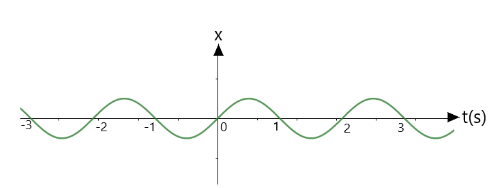Ans: Graph in figure (d), the motion of the particle repeats itself after 2s. Thus, it is a periodic motion with a period of 2s. 8. Which of the following relationships between the acceleration a and the displacement x of a particle involve simple harmonic motion? a) a=0.7x b) a=-200{{x}^{2}} c) a=-10x d) a=100{{x}^{3}} Ans: For SHM, acceleration and displacement are related by the relation (c) a=-10x (of the form a=-kx). 9. The acceleration due to gravity on the surface of moon is 1.7m/{{s}^{2}} . What is the time period of a simple pendulum on the surface of moon if its time period on the surface of earth is 3.5 s? (g on the surface of earth is 9.8m/{{s}^{2}}) Ans: In the above question it is given that: Acceleration due to gravity on the surface of moon is g'=1.7m/{{s}^{2}}. Acceleration due to gravity on the surface of earth is g=9.8m/{{s}^{2}}. Time period of a simple pendulum on earth is $T\text{ }=\text{ }3.5\text{ }s$. T=2\pi \sqrt{\frac{l}{g}} $l=\frac{{{T}^{2}}}{4{{\pi }^{2}}}g=\frac{{{\left( 3.5 \right)}^{2}}}{4{{\left( 3.14 \right)}^{2}}}\left( 9.8 \right)$ Where, l is the length of the pendulum. The length of pendulum remains constant On moon’s surface, time period, T'=2\pi \sqrt{\frac{l}{g'}} \Rightarrow T'=2\pi \sqrt{\frac{\frac{{{\left( 3.5 \right)}^{2}}}{4{{\left( 3.14 \right)}^{2}}}\left( 9.8 \right)}{1.7}}=8.4s Therefore, the time period of the simple pendulum on the surface of moon is 8.4s. 10. A simple pendulum of length l and having a bob of mass M is suspended in a car. The car is moving on a circular track of radius R with a uniform speed v. If the pendulum makes small oscillations in a radial direction about its equilibrium position, what will be its time period? Ans: From the above question it is clear that the bob of the simple pendulum will experience the acceleration due to gravity and the centripetal acceleration provided by the circular motion of the car. $\text{Acceleration due to gravity = g}$ $\text{Centripetal acceleration }{{\text{a}}_{c}}={{v}^{2}}/r$ where, v is the uniform speed of the car R is the radius of the track Hence, effective acceleration is given as: g'=\sqrt{{{g}^{2}}+{{a}_{c}}^{2}} g'=\sqrt{{{g}^{2}}+{{\left( \frac{{{v}^{2}}}{r} \right)}^{2}}} Time period, T=2\pi \sqrt{\frac{l}{g'}}\frac{dy}{dx} \Rightarrow T=2\pi \sqrt{\frac{l}{\sqrt{{{g}^{2}}+\frac{{{v}^{4}}}{{{r}^{2}}}}}} \Rightarrow T=2\pi \sqrt{\frac{l}{\sqrt{{{g}^{2}}+{{\left( \frac{{{v}^{2}}}{r} \right)}^{2}}}}}, which represents the time period here. 4 Marks Questions 1. Which of the following examples represent (nearly) simple harmonic motion and which represent periodic but not simple harmonic motion? a) The rotation of earth about its axis. Ans: When the earth rotates about its axis, it comes to the same position in fixed intervals of time. Hence, it is a periodic motion. However, earth does not have a to and fro motion about its axis. Hence, it is not a simple harmonic. b) Motion of an oscillating mercury column in a U-tube. Ans: In an oscillating mercury column in a U-tube, mercury moves to and from on the same path, about the fixed position, with a certain period of time. Hence, it is a simple harmonic motion. c) Motion of a ball bearing inside a smooth curved bowl, when released from a point slightly above the lowermost point. Ans: When a ball is released from a point slightly above the lowermost point, it moves to and from about the lowermost point of the bowl. Also, the ball comes back to its initial position in the fixed interval of time, again and again. Thus, this motion is periodic as well as simple harmonic. d) General vibrations of a polyatomic molecule about its equilibrium position. Ans: A polyatomic molecule possesses many natural frequencies of oscillation. Its vibration is the superposition of individual simple harmonic motions of a number of different molecules. Thus, it is not simple harmonic, but periodic. 2. Which of the following functions of time represent (a) simple harmonic, (b) periodic but not simple harmonic, and (c) non-periodic motion? Give period for each case of periodic motion ($\omega$ is any positive constant): a) \sin \omega t-\cos \omega t Ans: The given function is: \sin \omega t-\cos \omega t =\sqrt{2}\left[ \frac{1}{\sqrt{2}}\sin \omega t-\frac{1}{\sqrt{2}}\cos \omega t \right] =\sqrt{2}\left[ \sin \omega t\times \cos \frac{\pi }{4}-\cos \omega t\sin \frac{\pi }{4} \right] =\sqrt{2}\sin \left( \omega t-\frac{\pi }{4} \right) This function is SHM as it can be written in the form of a\sin \left( \omega t+\phi \right). Its period is 2\pi /\omega . b) {{\sin }^{3}}\omega t Ans: The given function is: {{\sin }^{3}}\omega t=\frac{1}{4}\left[ 3\sin \omega t-\sin 3\omega t \right] From the above equation it is clear that \sin \omega tand \sin 3\omega trepresent simple harmonic motion individually and hence does their combination. Hence, the given function is periodic but not simple harmonic. c) 3\cos \left( \pi /4-2\omega t \right) Ans: The given function is: 3\cos \left( \pi /4-2\omega t \right)=3\cos \left( 2\omega t-\pi /4 \right) This function represents simple harmonic motion because it can be written in the form aco\operatorname{s}\left( \omega t+\phi \right) Period =\frac{2\pi }{2\omega }=\frac{\pi }{\omega } d) \cos \omega t+\cos 3\omega t+\cos 5\omega t Ans: The given function is: \cos \omega t+\cos 3\omega t+\cos 5\omega t From the above equation it is clear that co\operatorname{s}\omega t, co\operatorname{s}2\omega tand co\operatorname{s}3\omega trepresent simple harmonic motion individually and hence does their combination. Hence, given function is periodic but not simple harmonic e) \exp \left( -{{\omega }^{2}}{{t}^{2}} \right) Ans: The given function is: \exp \left( -{{\omega }^{2}}{{t}^{2}} \right) which is an exponential function. As exponential functions do not repeat themselves, hence it is a non-periodic motion. f) 1+\omega t+{{\omega }^{2}}{{t}^{2}} Ans: The given function is: 1+\omega t+{{\omega }^{2}}{{t}^{2}} It is non-periodic. 3. A spring balance has a scale that reads from 0 to 50 kg. The length of the scale is 20 cm. A body suspended from this balance, when displaced and released, oscillates with a period of 0.6 s. What is the weight of the body? Ans: In the above question it is given that: Maximum mass that the scale can read is $M\text{ }=\text{ }50\text{ }kg$. Maximum displacement of the spring $=\text{ }Length\text{ }of\text{ }the\text{ }scale,\text{ }l\text{ }=\text{ }20\text{ }cm\text{ }=\text{ }0.2\text{ }m$ Time period, $T\text{ }=\text{ }0.6\text{ }s$ Maximum force exerted on the spring is $F\text{ }=\text{ }Mg$. where, $g\text{ }=\text{ }acceleration\text{ }due\text{ }to\text{ }gravity\text{ }=\text{ }9.8\text{ }m/{{s}^{2}}$ $F\text{ }=\text{ }50\text{ }\times \text{ }9.8\text{ }=\text{ }490\text{ }N$ ∴ Spring constant, k=\frac{F}{l}=\frac{490}{0.2}=2450N{{m}^{-1}}. Mass m, is suspended from the balance. Time period, T=t=2\pi \sqrt{\frac{m}{k}} \Rightarrow m=\left( \frac{T}{2\pi } \right)\times k={{\left( \frac{0.6}{2\times 3.14} \right)}^{2}}\times 2450=22.36kg Weight of the body $=\text{ }mg\text{ }=\text{ }22.36\times 9.8\text{ }=\text{ }219.167\text{ }N$ Therefore, the weight of the body is $219\text{ }N$. 4. Figures 14.29 correspond to two circular motions. The radius of the circle, the period of revolution, the initial position, and the sense of revolution (i.e., clockwise or anti- clockwise) are indicated on each figure.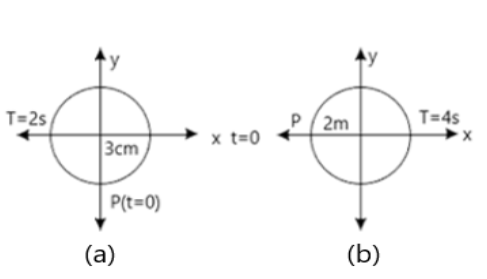Obtain the corresponding simple harmonic motions of the x-projection of the radius vector of the revolving particle P, in each case. Ans: a) In the figure (a) it is given that: Time period is $t\text{ }=\text{ }2\text{ }s$. Amplitude is $A\text{ }=\text{ }3\text{ }cm$. When, $t\text{ }=\text{ }0$ , the radius vector OP makes an angle \pi /2 with the positive x-axis. $Phase\text{ }angle=\pi /2$. Hence, the equation of simple harmonic motion for the x-projection of OP, at the time t, and is given by the displacement equation: x=A\cos \left[ \frac{2\pi t}{T}+\phi \right]=3\cos \left[ \frac{2\pi t}{2}+\frac{\pi }{2} \right]=-3\sin \left( \frac{2\pi t}{2} \right)cm \Rightarrow x=-3\sin \pi tcm, which represents the SHM equation here. b) In the figure (b) it is given that: Time Period, $t\text{ }=\text{ }4\text{ }s$. Amplitude, $a\text{ }=\text{ }2\text{ }m$. At time $t\text{ }=\text{ }0$, OP makes an angle \pi with the x-axis, in the anticlockwise direction, $Phase\text{ }angle=\pi$ Thus, the equation of simple harmonic motion for the x-projection of OP, at the time t, is x=A\cos \left[ \frac{2\pi t}{T}+\phi \right]=2\cos \left[ \frac{2\pi t}{4}+\pi \right] \Rightarrow x=-2\cos \left( \frac{\pi }{2}t \right)m, which represents the SHM equation here. 5. Cylindrical piece of cork of density of base area A and height h floats in a liquid of density ${{\rho }_{1}}$. The cork is depressed slightly and then released. Show that the cork oscillates up and down simple harmonically with a period T=2\pi \frac{h\rho }{{{\rho }_{1}}g}, where \rho is the density of cork. (Ignore damping due to viscosity of the liquid). Ans: In the above question it given that: $Base\text{ }area\text{ }of\text{ }the\text{ }cork\text{ }=\text{ }A$ $Height\text{ }of\text{ }the\text{ }cork\text{ }=\text{ }h$ Density of the liquid $={{\rho }_{1}}$ Density of the cork$=\rho$ In equilibrium: $Weight\text{ }of\text{ }the\text{ }cork\text{ }=\text{ }Weight\text{ }of\text{ }the\text{ }liquid\text{ }displaced\text{ }by\text{ }the\text{ }floating\text{ }cork$ If the cork is depressed slightly by x, some extra water of a certain volume is displaced. Hence, an extra up-thrust acts upward and provides the restoring force to the cork. $Upthrust\text{ }=\text{ }Restoring\text{ }force,\text{ }F\text{ }=\text{ }Weight\text{ }of\text{ }the\text{ }extra\text{ }water\text{ }displaced$ $F\text{ }=\text{ }\text{ }\left( Volume\text{ }\times \text{ }Density\text{ }\times \text{ }g \right)$ $Volume\text{ }=\text{ }Area\text{ }\times \text{ }Distance\text{ }through\text{ }which\text{ }the\text{ }cork\text{ }is\text{ }depressed$ \Rightarrow F=-Ax{{\rho }_{1}}g …… (i) According to the force law: F=kx\Rightarrow k=F/x where, k is constant. k=F/x=-A{{\rho }_{1}}g…… (ii) The time period of the oscillations of the cork: T=2\pi \sqrt{\frac{m}{k}}….. (iii) where, $m=\text{ }Mass\text{ }of\text{ }the\text{ }cork$ $m\text{ }=\text{ }Volume\text{ }of\text{ }the\text{ }cork\text{ }\times \text{ }Density$ $m\text{ }=\text{ }Base\text{ }area\text{ }of\text{ }the\text{ }cork\text{ }\times \text{ }Height\text{ }of\text{ }the\text{ }cork\text{ }\times \text{ }Density\text{ }of\text{ }the\text{ }cork$ $m=Ah\rho$ Hence, the expression for the time period becomes: T=2\pi \sqrt{\frac{Ah\rho }{A{{\rho }_{1}}g}}=2\pi \sqrt{\frac{h\rho }{{{\rho }_{1}}g}} 6. A mass attached to a spring is free to oscillate, with angular velocity \omega , in a horizontal plane without friction or damping. It is pulled to a distance {{x}_{0}} and pushed towards the centre with a velocity {{v}_{0}} at time t=0. Determine the amplitude of the resulting oscillations in terms of the parameters \omega ,{{x}_{0}} and {{v}_{0}}. ( Hint: Start with the equation x=a\cos \left( \omega t+\theta \right) and note that the initial velocity is negative.) Ans: In the above question it is given that: x=a\cos \left( \omega t+\theta \right) Where, A is the amplitude, x is the displacement, \theta is the phase constant. Then. $v=dx/dt=-A\omega \sin \left( \omega t+\theta \right)$ At t=0, x={{x}_{0}} {{x}_{0}}=A\cos \theta …… (i) And dx/dt=-{{v}_{0}}=A\omega \sin \theta A\sin \theta ={{v}_{0}}/\omega …… (ii) Squaring and adding (i) an (ii), {{A}^{2}}\left( {{\cos }^{2}}\theta +{{\sin }^{2}}\theta \right)={{x}_{0}}^{2}+\left( \frac{{{v}_{0}}^{2}}{{{\omega }^{2}}} \right) \Rightarrow A=\sqrt{{{x}_{0}}^{2}+{{\left( \frac{{{v}_{0}}}{\omega } \right)}^{2}}} Thus, the resulting amplitude is \sqrt{{{x}_{0}}^{2}+{{\left( \frac{{{v}_{0}}}{\omega } \right)}^{2}}}. 7. A spring having a spring constant $1200\text{ }N\text{ }{{m}^{1}}$ is mounted on a horizontal table as shown in figure. A mass of 3 kg is attached to the free end of the spring. The mass is then pulled sideways to a distance of 2.0 cm and released.Determine (i) the frequency of oscillations, (ii) maximum acceleration of the mass, and (iii) the maximum speed of the mass. Ans: In the above question it is given that: Spring constant is k=1200N{{m}^{-1}} Mass, $m\text{ }=\text{ }3\text{ }kg$ Displacement, $A\text{ }=\text{ }2.0\text{ }cm\text{ }=\text{ }0.02\text{ }cm$ i) Frequency of oscillation v, is given by: \upsilon =\frac{1}{T}=\frac{1}{2\pi }\sqrt{\frac{k}{m}} Here, T is the time period. \Rightarrow \upsilon =\frac{1}{2\pi }\sqrt{\frac{1200}{3}=3.18m/s} Thus, the frequency of oscillations is $3.18$ cycles per second. ii) Maximum acceleration (a) is given by: a={{\omega }^{2}}A Where, $\text{A= maximum displacement}$ \alpha =\frac{k}{m}A=\frac{1200\times 0.02}{3}=8m{{s}^{-2}} Thus, the maximum acceleration of the mass is 8m{{s}^{-2}}. iii) Maximum velocity is {{v}_{\max }}=A\omega =A\sqrt{\frac{k}{m}=0.02\times }\sqrt{\frac{1200}{3}=0.4m/s} Hence, the maximum velocity of the mass is 0.4 m/s. 8. Answer the following questions: a) Time period of a particle in SHM depends on the force constant k and mass m of the particle: T=2\pi \sqrt{\frac{m}{k}}. A simple pendulum executes SHM approximately. Why then is the time period of a pendulum independent of the mass of the pendulum? Ans: We know that: The time period of the simple pendulum is T=2\pi \sqrt{\frac{m}{k}} For a simple pendulum, k is expressed in terms of mass m, as: k\propto m, Where, \frac{m}{k} is a constant. Thus, the time period T, of a simple pendulum is independent of the mass of the bob. b) The motion of a simple pendulum is approximately simple harmonic for small angle oscillations. For larger angles of oscillation, a more involved analysis shows that T is greater than 2\pi \sqrt{\frac{l}{g}}. Think of a qualitative argument to appreciate this result. Ans: If we consider a simple pendulum, the restoring force acting on the bob of the pendulum is given by: $F=-mg\sin \theta$ Where, $\text{F = Restoring force}$ $\text{m = Mass of the bob}$ $\text{g = Acceleration due to gravity}$ $\theta \text{=Angle of displacement}$ For small \theta , \sin \theta \approx \theta For large \theta , \sin \theta >\theta This decreases the effective value of g. Thus, the time period increases as: T=2\pi \sqrt{\frac{l}{g}} where l is the length of the simple pendulum. c) A man with a wristwatch on his hand falls from the top of a tower. Does the watch give the correct time during the free fall? Ans: The time shown by the wrist watch of a man falling from the top of a tower is not affected by the fall. As a wristwatch does not work on the principle of a simple pendulum, it is not affected by the acceleration due to gravity during free fall. The working of wrist watch depends on spring action. d) What is the frequency of oscillation of a simple pendulum mounted in a cabin that is freely falling under gravity? Ans: If a simple pendulum mounted in a cabin falls freely under gravity, its acceleration is zero. Thus, the frequency of the oscillation of this pendulum is zero. 5 Marks Questions 1. What is simple pendulum? Find an expression for the time period and frequency of a simple pendulum. Ans: A simple pendulum consists of a heavy point mass body suspended by a weightless inextensible and perfectly flexible string from a rigid support which is free to oscillate. The distance between the point of suspension and the point of oscillation is the effective length of the pendulum. $M\text{ }=\text{ }Mass\text{ }of\text{ }Bob$ $x\text{ }=\text{ }Displacement\text{ }=\text{ }OB$ $l\text{ }=\text{ }length\text{ }of\text{ }simple\text{ }pendulum$Let the bob is displaced through a small angle θ the forces acting on it:- 1) $weight\text{ }=\text{ }Mg\text{ }acting\text{ }vertically\text{ }downwards$. 2) $Tension\text{ }=\text{ }T$ acting upwards. Dividing Mg into its components $mgcos\text{ }\theta \text{ }\And \text{ m}gsin\text{ }\theta$ $T\text{ }=\text{ m}gcos\text{ }\theta$ $F\text{ }=\text{ m}gsin\text{ }\theta$ - ve sign shows force is divested towards the ocean positions. If $\theta$ is Small, \sin \theta \approx \theta =\frac{A\text{rc OB}}{l}=\frac{x}{l} F=-mg\frac{x}{l} In S.H.M restoring force, -mg\sin \theta =-mg\theta =-\frac{mgx}{l} When, k=spring constant F=-kx -\frac{mgx}{l}=-kx k=\frac{mg}{l} T=2\pi \sqrt{\frac{m}{k}} T=2\pi \sqrt{\frac{ml}{mg}} T=2\pi \sqrt{\frac{l}{g}} Thus, f=\frac{1}{2\pi }\sqrt{\frac{g}{l}} 2. A particle is in linear simple harmonic motion between two points, A and B, 10 cm apart. Take the direction from A to B as the positive direction and give the signs of velocity, acceleration and force on the particle when it is Ans: Consider the figure given in the question. The two extreme positions, A and B are of a SHM. The positive direction of velocity is considered from A to B. The acceleration and the force along AP are positive and BP are negative.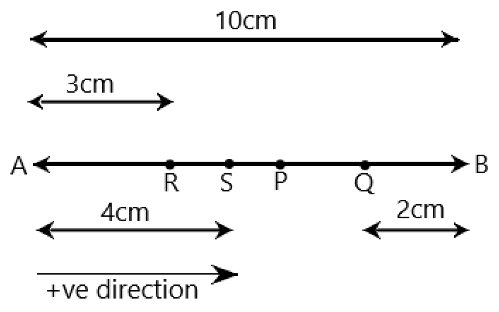a) At the end A, The particle that is executing SHM is momentarily at rest being its extreme position of motion at the end A. Hence, its velocity is zero. Acceleration is positive as it is directed along AP, force is also positive as the force is directed along AP. b) At the end B, Velocity is zero at the end B. As acceleration and force are directed along BP, hence they are negative. c) At the mid-point of AB going towards A, Along the direction towards A, at the midpoint of AB, the particle is at its mean position P and has a tendency to move along PA. Thus, velocity is positive. Both acceleration and force are zero. d) At 2 cm away from B going towards A, The position of particle at 2 cm away from B going towards A is at Q. At this position it has the tendency to move along QP, which is negative direction. Therefore, velocity, acceleration and force all are positive. e) At 3 cm away from A going towards B, The position of particle at 3 cm away from A going towards B is at R. It has a tendency to move along RP, which is a positive direction. Here, velocity, acceleration all are positive. f) At 4 cm away from B going towards A. The position of particle at 4 cm away from A going towards A, is at S. It has a tendency to move along SA, which is negative direction. Thus, velocity is negative but acceleration is directed towards mean position, along SP. Hence it is positive and also force is positive similarly. 3. The motion of a particle executing simple harmonic motion is described by the displacement function, x\left( t \right)=A\cos \left( \omega t+\varphi \right). If the initial $\left( \mathbf{t}\text{ }=\text{ }\mathbf{0} \right)$ position of the particle is 1 cm and its initial velocity is \omega cm/s, what are its amplitude and initial phase angle? The angular frequency of the particle is \pi {{s}^{-1}}. If instead of the cosine function, we choose the sine function to describe the SHM: x\left( t \right)=B\sin \left( \omega t+\alpha \right), what are the amplitude and initial phase of the particle with the above initial conditions? Ans: In the above question it is given that: Displacement at t=0, is x=1cm. Initial velocity, v=\text{ }\!\!\omega\!\!\text{ cm/sec}. We have, x\left( t \right)=A\cos \left( \omega t+\varphi \right) 1=A\cos \left( \omega \times 0+\varphi \right)=A\cos \varphi A\cos \varphi =1 ….. (i) Velocity, v=\frac{dx}{dt} \omega =-A\omega \sin \left( \omega t+\varphi \right) 1=A\sin \left( \omega \times 0+\varphi \right)=-A\sin \varphi A\sin \varphi =-1 …… (ii) Squaring and adding equations (i) and (ii), we get: {{A}^{2}}\left( {{\sin }^{2}}\varphi +{{\cos }^{2}}\varphi \right)=1+1$$\Rightarrow {{A}^{2}}=2$$\Rightarrow A=\sqrt{2}cm$

Dividing equation (ii) by equation (i), we get:

$\tan \varphi =-1$

$\Rightarrow \varphi =3\pi /4,7\pi /4.....$

SHM is given by:

$x\left( t \right)=B\sin \left( \omega t+\alpha \right)$

Substituting the given values in this equation, we get:

$1=B\sin \left[ \omega \times 0+\alpha \right]=1+1$

$B\sin \alpha =1$ …… (iv)

Squaring and adding equations (iii) and (iv), we get:

${{B}^{2}}\left( {{\sin }^{2}}\alpha +{{\cos }^{2}}\alpha \right)=1+1\Rightarrow {{B}^{2}}=2\Rightarrow B=\sqrt{2}cm$

Dividing equation (iii) by equation (iv), we get:

$\tan \alpha =1\Rightarrow \alpha =\pi /4,5\pi /4.....$

4. In Exercise 14.9, let us take the position of mass when the spring is unstretched as x = 0, and the direction from left to right as the positive direction of x-axis. Give x as a function of time t for the oscillating mass if at the moment we start the stopwatch (t = 0), the mass is

a) At the mean position,

b) At the maximum stretched position, and

c) At the maximum compressed position.

In what way do these functions for SHM differ from each other, in frequency, in amplitude or the initial phase?

Ans: In the above question it is given that:

Distance travelled by the mass sideways is $a\text{ }=\text{ }2.0\text{ }cm$.

Angular frequency of oscillation is given by:

$\omega =\sqrt{\frac{k}{m}=}\sqrt{\frac{1200}{3}=20rad/s}$

a) At the mean position

Ans: As time is noted from the mean position, the initial phase is $0$. Hence, Displacement, $x\text{ }=\text{ }2\text{ }sin\text{ }20\text{ }t$.

b) At the maximum stretched position

Ans: When the body is at maximum stretched position, it is at the extreme right position, with an initial phase of $\pi /2$ rad.

Then, displacement $x=\alpha \sin \left( \omega t+\frac{\pi }{2} \right)=\alpha \cos \omega t=2\cos 20t$.

c) At the maximum compressed position

Ans: The body is at the left position at maximum compressed position, with an initial phase of  $3\pi /2$ rad.

Then,

Displacement, $x=\alpha \sin \left( \omega t+\frac{3\pi }{2} \right)=-\alpha \cos \omega t=-2\cos 20t$

The functions differ in the initial phase. They neither differ in amplitude nor in frequency.

5. Plot the corresponding reference circle for each of the following simple harmonic motions. Indicate the initial (t = 0) position of the particle, the radius of the circle, and the angular speed of the rotating particle. For simplicity, the sense of rotation may be fixed to be anticlockwise in every case: (x is in cm and t is in s).

1. $x=-2\sin \left( 3t+\pi /3 \right)$

Ans: Given equation is

$x=-2\sin \left( 3t+\pi /3 \right)=-2co\operatorname{s}\left( 3t+\pi /3+\pi /2 \right)=-2co\operatorname{s}\left( 3t+5\pi /6 \right)$

If this equation is compared with the standard SHM equation

$x=A\cos \left[ \frac{2\pi t}{T}+\phi \right]$

Therefore, $A=2cm$,

$\phi =5\pi /6$,

$\omega =2\pi /t=3rad/s$

The motion of the particle is shown in fig (a).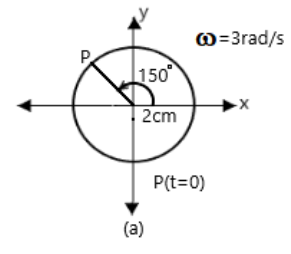1. $x=\cos \left( \pi /6-t \right)$

Ans: Given equation is

$x=\cos \left( \pi /6-t \right)=\cos \left( t-\pi /6 \right)$

If this equation is compared with the standard SHM equation

$x=A\cos \left[ \frac{2\pi t}{T}+\phi \right]$

Therefore, $A=1cm$,

$\phi =-\pi /6$,

$\omega =2\pi /t=1rad/s$

The motion of the particle is shown in fig (b).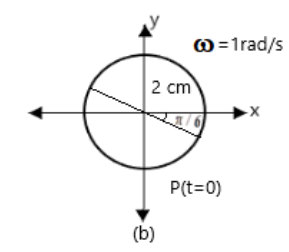6. Figure (a) shows a spring of force constant k clamped rigidly at one end and a mass m attached to its free end. A force F applied at the free end stretches the spring. Figure (b) shows the same spring with both ends free and attached to a mass m at either end. Each end of the spring in Fig. (b) is stretched by the same force F.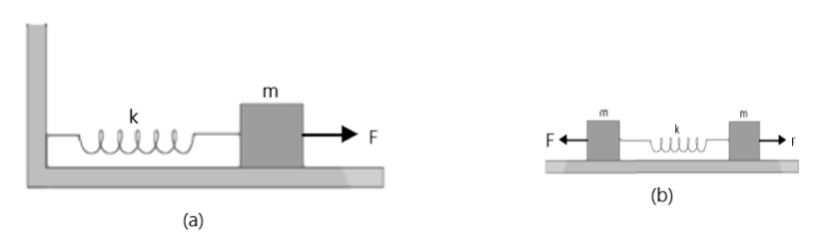a) What is the maximum extension of the spring in the two cases?

Ans: Consider one block system.

If a force F, is applied to the free end of the spring, then extension l, is produced. For the maximum extension,

i.e., $F=kl$

Where, k is the spring constant.

Thus, the maximum extension produced in the spring is $l=\frac{F}{k}$.

Now consider the two-block system:

In this case the displacement (x) produced is $x=\frac{l}{2}$.

Therefore, net force$=2kx=2k\left( \frac{l}{2} \right)$

$\Rightarrow l=\frac{F}{k}$

Thus, the maximum extension of the spring in both cases will be $\frac{F}{k}$.

b) If the mass in Fig. (a) and the two masses in Fig. (b) are released, what is the period of oscillation in each case?

Ans: In figure (a) if x is the extension in the spring, when mass m is returning to its mean position after being released free, then restoring force on the mass is $F=-kx$, i.e., $F\propto x$. As, this F is directed towards the mean position of the mass, hence the mass attached to the spring will execute SHM.

Spring factor = spring constant = k

inertia factor = mass of the given mass = m

As time period,

$T=2\pi \sqrt{\frac{\text{inertia factor}}{\text{spring factor}}}=2\pi \sqrt{\frac{m}{k}}$

If we consider figure (b), we have a two-body system of spring constant k and reduced mass,

$\mu =\frac{m\times m}{m+m}=\frac{m}{2}$

$Inertia\text{ }factor\text{ }=\text{ }m/2$

$Spring\text{ }factor\text{ }=\text{ }k$

Hence, time period, $T=2\pi \sqrt{\frac{m/2}{k}=}2\pi \sqrt{\frac{m}{2k}}$.

7. One end of a U-tube containing mercury is connected to a suction pump and the other end to the atmosphere. A small pressure difference is maintained between the two columns. Show that, when the suction pump is removed, the column of mercury in the U-tube executes simple harmonic motion.

Ans: Consider,

$Area\text{ }of\text{ }cross-section\text{ }of\text{ }the\text{ }U-tube\text{ }=\text{ }A$

$Density\text{ }of\text{ }the\text{ }mercury\text{ }column\text{ }=\rho$

$\text{Acceleration due to gravity = g}$

$\text{Restoring force, F = Weight of the mercury column of a certain height}$

$F\text{ }=\text{ }\left( Volume\text{ }\times \text{ }Density\text{ }\times \text{ }g \right)$

$F=-\left( 2A\times h\times \rho \times g \right)=-2A\rho gh$

$=k\times \text{Displacement in one of the arms (h)}$

Where,

$2h$ is the height of the mercury column in the two arms

k is a constant, given by

$k=-F/h=2A\rho g$

Time period, $T=2\pi \sqrt{\frac{m}{A\rho g}}$

where,

m is the mass of the mercury column

Let l be the length of the total mercury in the U-tube

$Mass\text{ }of\text{ }mercury,\text{ }m\text{ }=\text{ }Volume\text{ }of\text{ }mercury\text{ }\times \text{ }Density\text{ }of\text{ }mercury=Al\rho$

$T=2\pi \sqrt{\frac{Al\rho }{2A\rho g}}=2\pi \sqrt{\frac{l}{2g}}$

Thus, the mercury column executes simple harmonic motion with time period $2\pi \sqrt{\frac{l}{2g}}$.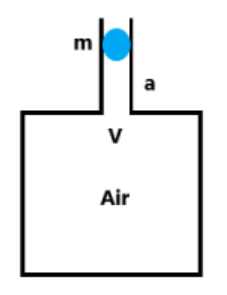a) $x=3\sin \left( 2\pi t+\pi /4 \right)$

Ans: Given equation is

$x=3\sin \left( 2\pi t+\pi /4 \right)=-3\cos \left( 2\pi t+\pi /4+\pi /2 \right)=-3\cos \left( 2\pi t+3\pi /4 \right)$

If this equation is compared with the standard SHM equation

$x=A\cos \left[ \frac{2\pi t}{T}+\phi \right]$

Therefore, $A=3cm$,

$\phi =3\pi /4$,

$\omega =2\pi /t=2rad/s$

The motion of the particle is shown in fig (c).

(Image Will Be Updated Soon)

b) $x=2\cos \pi t$

Ans: Given equation is

$x=2\cos \pi t$

If this equation is compared with the standard SHM equation

$x=A\cos \left[ \frac{2\pi t}{T}+\phi \right]$

Therefore, $A=2cm$,

$\phi =0$,

$\omega =\pi rad/s$

The motion of the particle is shown in fig (d).

(Image Will Be Updated Soon)

8. An air chamber of volume V has a neck area of cross section ‘a’ into which a ball of mass ‘m’ just fits and can move up and down without any friction (Fig.14.33). Show that when the ball is pressed down a little and released, it executes SHM. Obtain an expression for the time period of oscillations assuming pressure-volume variations of air to be isothermal (see figure).

(Image Will Be Updated Soon)

Ans: In the above question it given that:

$Volume\text{ }of\text{ }the\text{ }air\text{ }chamber=V$

$Area\text{ }of\text{ }cross-section\text{ }of\text{ }the\text{ }neck\text{ }=\text{ }a$

$Mass\text{ }of\text{ }the\text{ }ball\text{ }=\text{ }m$

$The\text{ }pressure\text{ }inside\text{ }the\text{ }chamber=atmospheric\text{ }pressure.$

Let the ball be depressed by x units. As a result of this depression, there would be a decrease in the volume and an increase in the pressure inside the chamber.

Decrease in the volume of the air chamber, $\Delta V=ax$

$\text{Volumetric Strain = }\frac{\text{change in volume}}{\text{original volume}}=\frac{ax}{V}$

Bulk modulus of air, $B=\frac{Stress}{Strain}=\frac{-p}{\frac{ax}{V}}$

Here, stress is the increase in pressure. The negative sign indicates that pressure increases with decrease in volume.

$p=-Bax/V$

The restoring force acting on the ball,

$F=p\times a$

$=-\frac{B{{a}^{2}}x}{V}$ …… (i)

In simple harmonic motion, the equation for restoring force is:

$F\text{ }=\text{ }-kx$ ..... (ii)

where, k is the spring constant

Comparing equations (i) and (ii), we get:

$k=-\frac{B{{a}^{2}}}{V}$

Time Period,

$T=2\pi \sqrt{\frac{m}{k}}=2\pi \sqrt{\frac{Vm}{B{{\alpha }^{2}}}}$

9. You are riding in an automobile of mass 3000 kg. Assuming that you are examining the oscillation characteristics of its suspension system. The suspension sags 15 cm when the entire automobile is placed on it. Also, the amplitude of oscillation decreases by 50% during one complete oscillation. Estimate the values of

a) the spring constant k

Ans: In the above question it is given that:

$Mass\text{ }of\text{ }the\text{ }automobile,\text{ }m\text{ }=\text{ }3000\text{ }kg$

$Displacement\text{ }in\text{ }the\text{ }suspension\text{ }system,\text{ }x\text{ }=\text{ }15\text{ }cm\text{ }=\text{ }0.15\text{ }m$

There are 4 springs in parallel to the support of the mass of the automobile.

The equation for the restoring force for the system is $F\text{ }=\text{ }4kx\text{ }=\text{ }mg$.

Where, k is the spring constant of the suspension system.

Time period, $T=2\pi \sqrt{\frac{m}{4k}}$

And

$k=mg/4x=3000\times 10/4\times 0.15=5000=5\times {{10}^{4}}Nm$

Spring Constant, $k=5\times {{10}^{4}}Nm$.

b) The damping constant b for the spring and shock absorber system of one wheel, assuming that each wheel supports 750 kg.

Ans: We know that each wheel supports a mass, $M\text{ }=\text{ }3000/4\text{ }=\text{ }750\text{ }kg$.

For damping factor b, the equation for displacement is written as:

$x={{x}_{0}}{{e}^{-bt/2M}}$

The amplitude of oscillation decreases by $50\text{ }%$.

$x={{x}_{0}}/2$

$\Rightarrow {{x}_{0}}/2={{x}_{0}}{{e}^{-bt/2M}}$

${{\log }_{e}}2=bt/2M$

$b=2M{{\log }_{e}}2/t$

Where,

$T=2\pi \sqrt{\frac{m}{4k}}=2\pi \sqrt{\frac{3000}{4\times 5\times {{10}^{4}}}}=0.7691s$

$\Rightarrow b=\frac{2\times 750\times 0.693}{0.7691}=1351.53kg/s$

Hence, the damping constant of the spring is $1351.53kg/s$.

10. Show that for a particle in linear SHM the average kinetic energy over a period of oscillation equals the average potential energy over the same period.

Ans: Consider,

The equation of displacement of a particle executing SHM at an instant t is given as:

$x=A\sin \omega t$

where,

A is the amplitude of oscillation,

$\text{Angular frequency=}\omega \text{=}\sqrt{\frac{k}{M}}$

The velocity of the particle is given by $v=dx/dt=A\omega \cos \omega t$.

The kinetic energy of the particle is:

${{E}_{k}}=1/2M{{v}^{2}}=1/2M{{A}^{2}}{{\omega }^{2}}{{\cos }^{2}}\omega t$

The potential energy of the particle is:

${{E}_{p}}=1/2k{{x}^{2}}=1/2{{M}^{2}}{{\omega }^{2}}{{A}^{2}}{{\sin }^{2}}\omega t$

For time period T, the average kinetic energy over a single cycle is given as:

${{\left( {{E}_{k}} \right)}_{Avg}}=\frac{1}{T}\int\limits_{0}^{T}{{{E}_{k}}dt}$

$=\frac{1}{T}\int\limits_{0}^{T}{\frac{1}{2}M{{A}^{2}}{{\omega }^{2}}co{{s}^{2}}\omega tdt}$

$=\frac{1}{2T}M{{A}^{2}}{{\omega }^{2}}\left[ 1+\frac{\sin 2\omega t}{2\omega } \right]_{0}^{T}$

$=\frac{1}{2T}M{{A}^{2}}{{\omega }^{2}}\left( T \right)$

$=\frac{1}{4}M{{A}^{2}}{{\omega }^{2}}$ ……. (i)

And, average potential energy over one cycle is given as:

${{\left( {{E}_{p}} \right)}_{Avg}}=\frac{1}{T}\int\limits_{0}^{T}{{{E}_{p}}dt}$

$=\frac{1}{T}\int\limits_{0}^{T}{\frac{1}{2}M{{A}^{2}}{{\omega }^{2}}{{\sin }^{2}}\omega tdt}$

$=\frac{1}{2T}M{{\omega }^{2}}{{A}^{2}}\int\limits_{0}^{T}{\frac{\left( 1+\cos 2\omega t \right)}{2}}dt$

$=\frac{1}{4T}M{{\omega }^{2}}{{A}^{2}}\left( T \right)$

$=\frac{M{{\omega }^{2}}{{A}^{2}}}{4}$ …… (ii)

It can be interpreted from equations (i) and (ii) that the average kinetic energy for a given time period is equal to the average potential energy for the same time period.

11. A body describes simple harmonic motion with amplitude of 5 cm and a period of 0.2 s. Find the acceleration and velocity of the body when the displacement is (a) 5 cm, (b) 3 cm, (c) 0cm.

Ans: In the above question it is given that:

Amplitude, r= 5 cm = 0.05 m

$r=5cm=0.05m$

Time period, $T\text{ }=\text{ }0.2\text{ }s$.

$\omega =2\pi /T=2\pi /0.2=1\text{0 }\!\!\pi\!\!\text{ rad/s}$

When displacement is y, then acceleration, $A=-{{\omega }^{2}}y$.

Velocity is $V=\omega \sqrt{{{r}^{2}}-{{y}^{2}}}$

Now,

Case (a):

When $y\text{ }=\text{ }5cm\text{ }=\text{ }0.05\text{ }m$

$A=-{{\left( 10\pi \right)}^{2}}\times 0.05=-5{{\pi }^{2}}m/{{s}^{2}}$

$V=10\pi \sqrt{{{\left( 0.05 \right)}^{2}}-{{\left( 0.05 \right)}^{2}}}=0$

Case (b):

When $y\text{ }=\text{ 3}cm\text{ }=\text{ }0.03\text{ }m$

$A=-{{\left( 10\pi \right)}^{2}}\times 0.03=-3{{\pi }^{2}}m/{{s}^{2}}$

$V=10\pi \sqrt{{{\left( 0.05 \right)}^{2}}-{{\left( 0.03 \right)}^{2}}}=0.4\pi m/s$

Case (c):

When $y\text{ }=\text{ 0}cm\text{ }=\text{ }0\text{ }m$

$A=0m/{{s}^{2}}$

$V=10\pi \sqrt{{{\left( 0.05 \right)}^{2}}-{{\left( 0 \right)}^{2}}}=0.5\pi m/s$

where, in each case, A represents the required acceleration and V represents the required velocity.